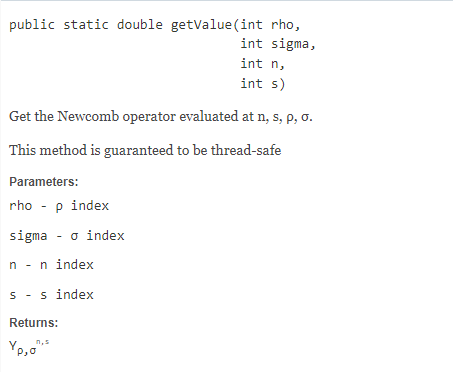# Theory behind modified Newcomb operators computation

Hi everyone,

It might not be a matter of interest but I am currently doing my master’s degree thesis and I’ve been obtaining some results from the DSSTPropagation tutorial class.
I was analyzing the implementation of the semi-analytical theory in these codes and I was wondering if there’s any reference that supports the method followed to calculate the modified Newcomb coefficients.

I’ve consulted Danielson’s paper of Semianalytic Satellite Theory but I haven’t found anything linked with the polynomial decomposition that’s performed in Orekit’s code. I would really appreciate any suggestion.

Laura

I think @lucian explained it in the following paper: On the Computation of the Hansen Coefficients

But my doubt is more related with the advantages of storing the Newcomb operators as an array of Polynomial Function (I attach a screenshot of the description of NewcombOperators Class ).

I think the storage is implemented in order to avoid regenerating the operators each time.
It could be seen as an implementation detail, and should give the same result as applying the recurrence relation each time.

Yes, I see that, but I was talking about the definition of P_{kj} polynomial.

As far as I’ve been able to understand, Newcomb operators are the result of multiplying the elements of P_{kj} array by n powers (as many as elements stored in P_{kj}) and gradually adding each one of them. However, I don’t see which are the advantages of substituting the “n” and “s” values at the end of the computation (through the “getValue” method) instead of doing it from the beginning, avoiding to create the P_{kj} polynomial array as a function of s.

Sorry for the inconveniences, I’m aware my English is not really good and maybe I haven’t expressed myself clearly.Hi Iam,

Good questions, but I’m not sure the developers have an answer for you other than that is the way it was written. Is there a better way to write that code? Either from an accuracy or performance perspective? Perhaps you’re trying to imply Horner’s method should be used to evaluate the polynomial? Having a test case that compares what you think the implementation should be with the current implementation would help support your point.

Best Regards,
Evan

Hi Evan,

Thank you for your answer. No, I don’t know a better way to write it, it’s just because it took me a bit to understand the way of the polynomial array is created so I wondered if there was a paper where this method was explained.

Sorry for the possible inconveniences.

No inconveniences, discussion is good.Hi again!I don’t want to be a burden but while I was trying to understand the way Newcomb operators are computed I’ve found a possible error.

I was trying to obtain the corresponding Newcomb operator to the index set (n = -9, s = -10, rho = 11, sigma = 0). According to equation 2.7.3 -(12) of Danielson’s paper, since sigma is equal to 0, it only requires two set of Newcomb operators: (n = -9, s = -9, rho = 10, sigma = 0) and (n = -9, s = -8, rho = 9, sigma = 0) multiplied by its corresponding “recurrent coefficients” 2*(2*s-n) and (s-n) respectively.

Once each of these terms are multiplied, we compute the sum of them and then, we divide this result by 4*(sigma+rho).

Taking into account that:
Y (n = -9, s = -9, rho = 10, sigma = 0) = -2.41226E-09 (provided by NewcombOperator’s method, getValue)
Y (n = -9, s = -8, rho = 9, sigma = 0) = 5.36605E-09 (provided by NewcombOperator’s method, getValue)
2*(2s-n)/4(sigma+rho) = -0.5
(s-n)/4*(sigma+rho) = -0.022727273

The obtained Newcomb operator for the set (n = -9, s = -10, rho = 11, sigma = 0) is: 1.08E-09
but the result provided by Orekit’s code is: 1.19E-09.

Probably I am making a mistake somewhere but I don’t see it. I would really appreciate any explanation. Thanks in advance.